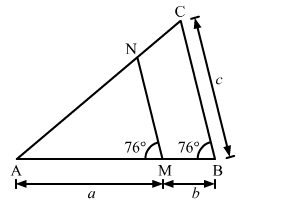# In the given figure, ∠AMN = ∠MBC = 760 If p, q and r are the lengthsQuestion:

In the given figure, ∠AMN = ∠MBC = 760 If pq and r are the lengths of AM, MB and BC respectively, then express the length of MN  in terms of pq and r.Solution:

In △AMN and △ABC
∠AMN = ∠ABC = 760     (Given)
∠A = ∠A         (Common)
By AA similarity criterion, △AMN ∼ △ABC
If two triangles are similar, then the ratio of their corresponding sides are proportional

$\therefore \frac{\mathrm{AM}}{\mathrm{AB}}=\frac{\mathrm{MN}}{\mathrm{BC}}$

$\Rightarrow \frac{\mathrm{AM}}{\mathrm{AM}+\mathrm{MB}}=\frac{\mathrm{MN}}{\mathrm{BC}}$

$\Rightarrow \frac{a}{a+b}=\frac{\mathrm{MN}}{c}$

$\Rightarrow \mathrm{MN}=\frac{a c}{a+b}$